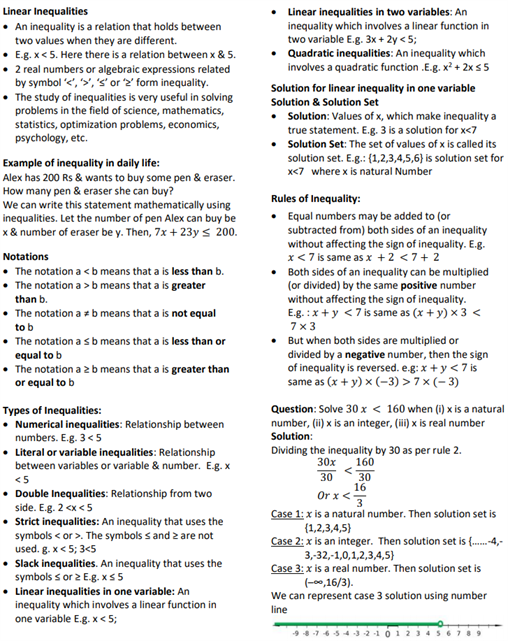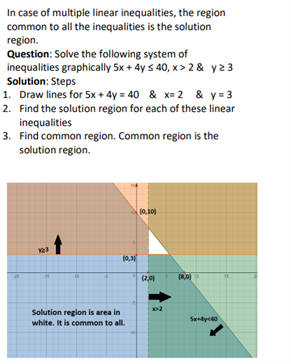# Revision Notes For CBSE Class 11 Maths Chapter 6 Linear Inequalities

Here students can find the class 11 maths revision notes Chapter 6 Linear Inequalities. Students will find the definition of all the important topics in maths such as types of inequalities, rules of inequalities etc.

For your convenience, we have shown you the procedure to solve a linear inequality

• Simplify both sides by removing group symbols and collecting like

terms.

• Remove fractions by multiplying both sides by an appropriate

factor.

• Isolate all variable terms on one side and all constants on the

other side.

• Make the coefficient of variable 1 and get the solution

(whenever coefficient of x is negative multiply throughout by -1 so

that the inequality is reversed.

Eg -2x ≥ 5

2x ≤ -5

x ≤-5/2

x ≤ -2.5 therefore solution is (-∞,-2.5]

Note: If x≥ 0, y≥0 is given in question every point in the shaded

region in the first quadrant including the points on the line and the

axis represents the solution of the given system of inequalities.

They will also see the definition of numerical, literal, double, strict, slack types of inequalities etc. along with that, they will be exposed to a graph showing the graphical solution of linear inequalities in 2 variables. These notes will be very helpful to students who can find all the important concepts related to this chapter.

Find the class 11 maths revision notes Chapter 6 Linear Inequalities here.